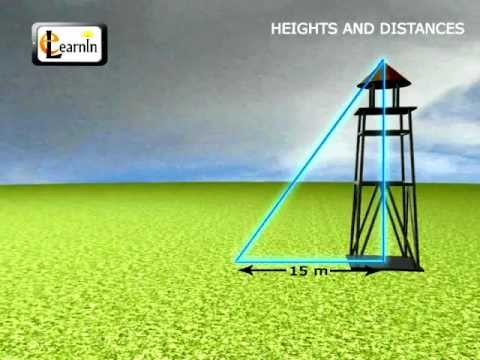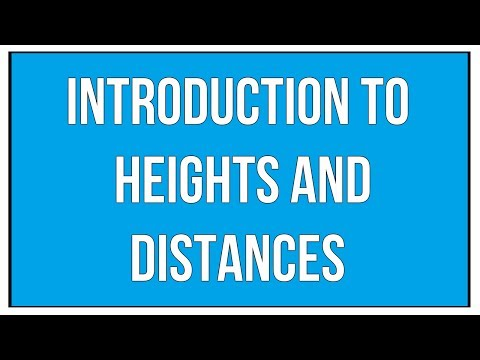## Height and distance

Subject: Compulsory Mathematics

#### Overview

The angle made by the line through his eyes and top of the tree with the line parallel to the ground is called the angle of elevation. The angle formed by the line joining the object and the eye of the observer with the horizon is called the angle of depression.

### Angle of Elevation

In the figure given below, a man, standing on the ground in front of a tree, is looking at the top of the tree. The angle made by the line through his eyes and top of the tree with the line parallel to the ground is called the angle of elevation.When the observer looks at an object above him, the angle formed by the line joining the object and the eye of the observer with the ground or parallel to the ground is called the angle of elevation.

### Angle of depression

When an observer observes an object below him, the angle formed by the line joining the object and the eye of the observer with the horizon is called the angle of depression.

In the figure given below a man, on the rooftop of the house, is looking a car parked on the roadside in front of his house. He finds an angle made by the line through his eye and the point of the car with the horizon. Such angle is called the angle of depression.##### Things to remember

When the observer looks at an object above him, the angle formed by the line joining the object and the eye of the observer with the ground or parallel to the ground is called the angle of elevation.

When an observer observes an object below him, the angle formed by the line joining the object and the eye of the observer with the horizon is called the angle of depression.

• It includes every relationship which established among the people.
• There can be more than one community in a society. Community smaller than society.
• It is a network of social relationships which cannot see or touched.
• common interests and common objectives are not necessary for society.
##### Videos for Height and distance##### Heights and Distances - Trigonometry - Maths##### Introduction To Heights and Distances / Maths##### Trigonometry Height and Distance Type 1##### Trigonometry Height and Distance Type 2##### Trigonometry Height and Distance Type 3##### Trigonometry Height and Distance Type 4##### Trigonometry Height and Distance Type 5##### Trigonometry Height and Distance Type 6

Suppose, AC denotes the tower, $\theta$ denotes the angle of elevation and AB denotes the distance between the man and the tower.

Then,

AC = 80$\sqrt 3$ m

$\angle$ABC = $\theta$

AB = 240 m

In $\triangle$ABC,

\begin{align*} tan \theta &= \frac{AC}{AB}\\ &= \frac{80\sqrt 3 m}{240 m}\\ &= \frac{\sqrt 3}{3}\\ &= \frac1{\sqrt 3}\\ \end{align*}

Then,

tan $\theta$ = tan 30°

∴ $\theta$ = 30°Ans

Suppose,

AB is the tower and AC is sun's ray. AC makes an angle of 30° with the ground.

AB =m 50m

BC is the shadow of the tower.

In $\triangle$ABC,

tan 30° = $\frac {AB}{CB}$

or, $\frac1{\sqrt 3}$ = $\frac {50 m}{BC}$

or, BC = 50× $\sqrt 3$ m

∴ BC = 86.60 mAns

Let AC be the length of the broken part of the tree and AB be the vertical part of the tree. Let AC make 60° with CB i.e. $\angle$ ACB = 60°. Suppose AC = x then AB = (14 - x)m.

In right angled $\triangle$ACB,

sin $\angle$ACB = $\frac{AB}{AC}$

or, sin 60° = $\frac {14 - x}{x}$

or, $\frac {\sqrt 3}{2}$ = $\frac {14 - x}{x}$

or, $\sqrt 3$ x = 28 - 2x

or, $\sqrt 3$ x + 2x = 28

or, x ($\sqrt 3$ + 2) = 28

or, x (1.73 + 2) = 28

or, x× 3.73 = 28

or, x = $\frac {28}{3.73}$

∴ x = 7.4 mAns

Let AC be the length of the broken part of the tree and AB be the vertical part of the tree.

Let AC makes an angle of 60° with CB i.e. $\angle$ACB = 60°.

Suppose AC = x.

Here,

CB = 15$\sqrt 3$

Now,

In the right angled $\triangle$ABC,

cos 60° = $\frac {CB}{AC}$

or, $\frac 12$ = $\frac {15\sqrt 3}{AC}$

or, AC = x = 30$\sqrt 3$

Again,

tan 60° = $\frac {AB}{CB}$

or, $\sqrt 3$ = $\frac {AB}{15\sqrt 3}$

or, AB = 15$\sqrt 3$× $\sqrt 3$

∴ AB = 45

Hence, height of the tree before it was broken = AB + AC = 30$\sqrt 3$ + 45 = 51.96 + 45 = 96.96 m.

∴ The total height of the tree is 96.96 m.Ans

Let AB be the height of the man and CD be the pole. Let AE be the horizontal line which is parallel to

the ground BD.

Here,

BD = 86 m

CD = 52 m

$\angle$CAE = 30°

AB = ?

From $\triangle$ACE,

tan 30° = $\frac{CE}{AE}$ = $\frac{CE}{86 m}$ [$\because$ AE = BD = 86 m]

or, $\frac1{\sqrt 3}$ = $\frac{CE}{86 m}$

or, CE = $\frac{86 m}{\sqrt 3}$

∴ CE = 49.65 m

Now,

ED = CD - CE = 52 m - 49.65 m = 2.35 m

∴ AB = ED = 2.35 m

Hence, height of the man is 2.35 m.Ans

Let AB be the house and CD be the tower. The distance between house and tower in BD = 20$\sqrt 3$ m and AE is horizontal line.

Here,

AB = 8 m

AE = BD = 20$\sqrt 3$

$\angle$CAE = 60°

In right angled $\triangle$AEC,

tan 60° = $\frac{CE}{AE}$

or, $\sqrt 3$ = $\frac{CE}{20\sqrt 3}$

or, CE = 20 ($\sqrt 3$)2

∴ CE = 60 m

Now,

ED = AB = 8 m

∴ CD = CE + ED = 60 m + 8 m = 68 m

Hence, the height of the tower is 68 m.Ans

Suppose, AB be a boy and CD be the height of kite from the ground. AD is thread.

Here,

AB = 1.8 m

$\angle$DAE = 60°

EC = AB =1.8 m

From right angled $\triangle$DAE,

sin 60° = $\frac{DE}{AD}$

or, $\frac{\sqrt 3}{2}$ = $\frac{DE}{300 m}$

or, DE = $\frac {300 m × \sqrt 3}{2}$

∴ DE = 259.80 m

Hence, the height of the kite from the ground = CD = DE + EC = 259.80 m + 1.8 m = 261.60 mAns

Suppose, OA is pole, O is the centre of circular pond, OB is a radius r of the pond and $\angle$ABO is angle of elevation.

Here,

OB = r

$\angle$ABO = 60°

OA =?

By question,

Circumference = 176 m

or, 2$\pi$r = 176 m

or, r = $\frac{176 × 7}{2 × 22}$m

∴ r = 28 m

From $\triangle$AOB,

tan 60° = $\frac{AO}{BO}$

or, $\sqrt 3$ = $\frac {AO}r$

or, AO =r$\sqrt 3$

or, AO = 28× $\sqrt 3$

∴ AO = 48.49 m

Hence, height of the pole is 48.49 m.Ans

Let AB be the man and DC be the house. BC denotes the distance between the man and house. AE is a horizontal line.

Here,

AB = 1.6 m

BC = 100 m

EC = AB = 1.6 m

AE = BC = 100 m

$\angle$DAE = 60°

From right angled $\triangle$AED,

tan 60° = $\frac{DE}{AE}$

or, $\sqrt 3$ = $\frac {DE}{100 m}$

or, DE = $\sqrt 3$× 100 m

∴ DE = 173.21 m

Here,

EC = AB = 1.6 m

Hence, height of the house = DE + EC = 173.21 m + 1.6 m = 174.81 mAns

Here,

Height of the tower (PQ) = ?

Distance between R and S (RS) = 30m

Angle of elevation ($\angle$ PRQ) = 45°

Angle of elevation ($\angle$ PSQ) = 30°

We have,

In right angled triangle $\triangle$ PQR:

tan 45° = $\frac {PQ}{PR}$

1 = $\frac {PQ}{PR}$

∴ PR = PQ

In right angled triangle $\triangle$ PQS:

tan 30° = $\frac {PQ}{PS}$

or, $\frac 1{\sqrt 3}$ = $\frac {PQ}{30 + PR}$ [$\because$ PQ = PR]

or, 30 + PQ = $\sqrt 3$ PQ

or, $\sqrt 3$ PQ - PQ = 30

or, PQ ($\sqrt 3$ - 1) = 30

or, PQ = $\frac {30}{\sqrt 3 - 1}$

or, PQ = $\frac {30}{1.732 - 1}$

or, PQ = $\frac {30}{0.732}$

∴ PQ = 40.98 m

∴ The height of tower (PQ) = 40.98 m Ans